Author: Oscar Cronquist Article last updated on January 22, 2019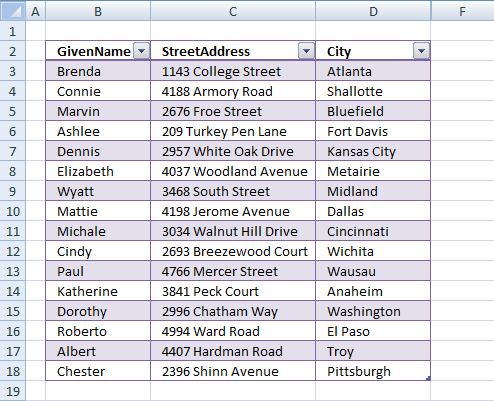This article demonstrates how to quickly compare two data sets in Excel using a formula and Excel defined Tables. The formula will return TRUE if a record is found in the other data set and FALSE if not.

The image above shows you the first data set: Table1 The image below shows you the second data set: Table2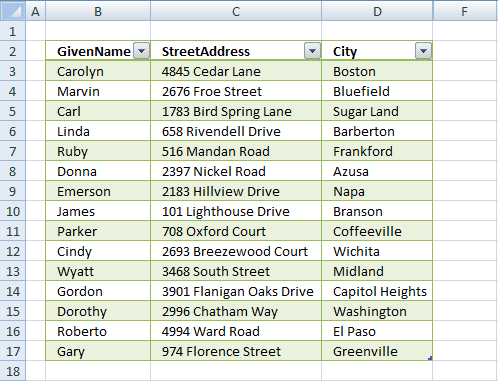Excel defined tables has many advantages, one is that you only need to enter a formula in one cell and Excel automatically enters the formula in the remaining cells in the same column.

Another one is that it expands accordingly when new data is added, no need to adjust the cell references. You can also easily filter the data using the arrows in the top row of the Excel table.

How to convert a data set to an Excel defined Table:

1. Select a cell in the data set.
2. Press CTRL + T.
3. Click checkbox if your data set has headers.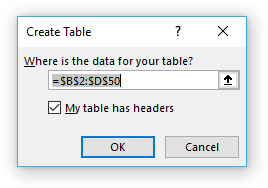4. Click OK.

Type the following formula in the next column adjacent to the Excel defined Table:

=COUNTIFS(Table2[GivenName],[@GivenName],Table2[StreetAddress],[@StreetAddress],Table2[City],[@City])>0

The Excel defined Table will automatically expand shown in the image below and enter the formula in all cells below in that column.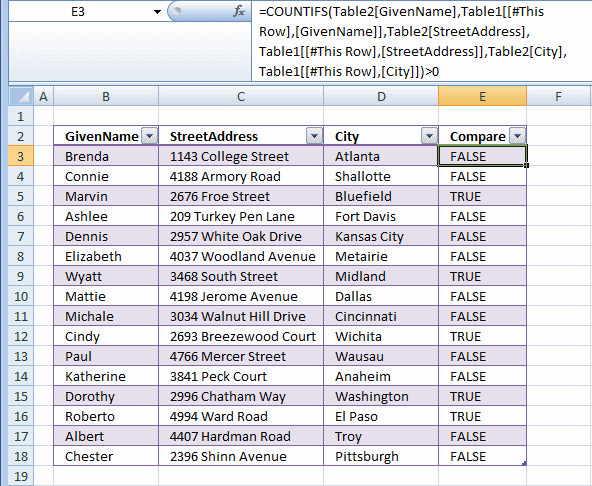### Explaining formula in cell E3

The COUNTIFS function calculates the number of cells across multiple ranges that equals all given conditions. There is a criteria range and a condition forming a pair, our Excel table has three columns so wee need three pairs in order to find matching records in the other table.

COUNTIFS(criteria_range1criteria1, [criteria_range2criteria2]…)

#### Step 1 - Build COUNTIFS function

The first argument is a cell reference to the entire column in table2 and the second argument is a cell reference to the cell on the same row as the formula but in column GivenName. Excel automatically enters these cell references (structured references) if you select the cells with your mouse when you build the formula.

You can also type the table name and column name in brackets, the formula bar will guide you in this process.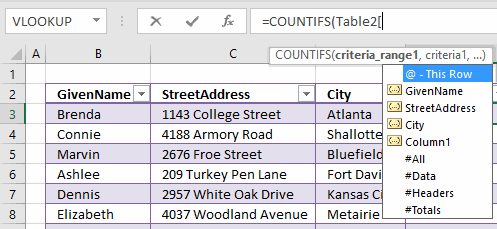COUNTIFS(Table2[GivenName],[@GivenName]

Complete the function by adding the remaining cell references to the COUNTIFS function.

COUNTIFS(Table2[GivenName],[@GivenName],Table2[StreetAddress],[@StreetAddress],Table2[City],[@City])

#### Step 2 - Logical expression

The COUNTIFS function returns a number representing how many records that match the current record in the other table. A number larger than zero indicates that there is at least one match.

COUNTIFS(Table2[GivenName],[@GivenName],Table2[StreetAddress],[@StreetAddress],Table2[City],[@City])>0

TRUE - At least one match.

FALSE - No match.

### Filter records occuring in both tables

1. Select sheet 1 (Table1)
2. Click black arrow in column Compare
3. Disable FALSE
4. Click OK.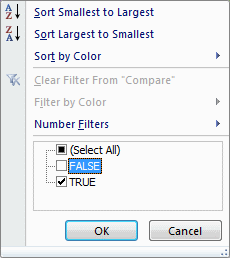The image below shows a filtered table with records that exists in both tables.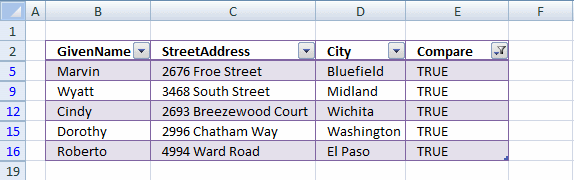### Filter records existing in only one table

1. Select a sheet
2. Click black arrow in column Compare
3. Enable only FALSE
4. Click OK.

Table1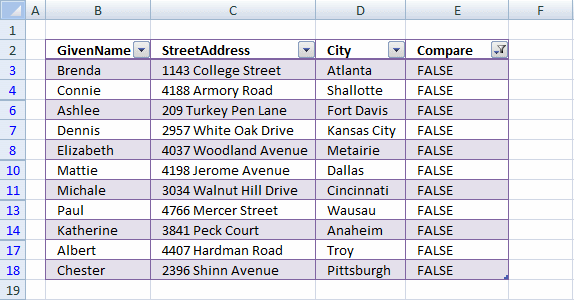Table2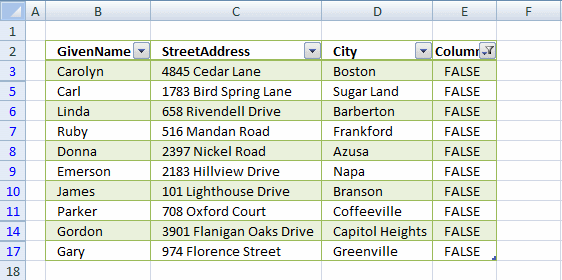### Download Excel file

* You will also get a weekly newsletter, unsubscribe anytime!